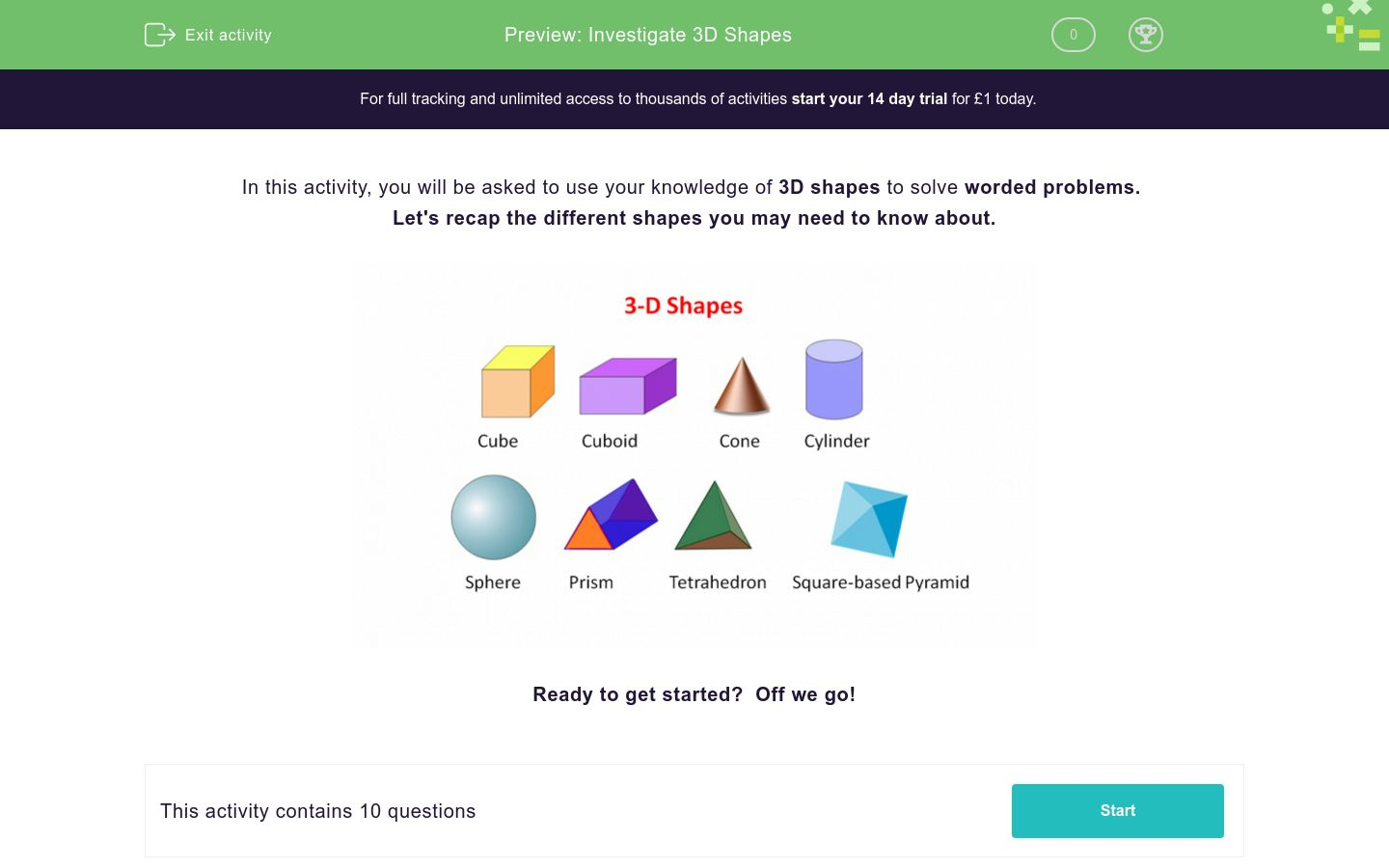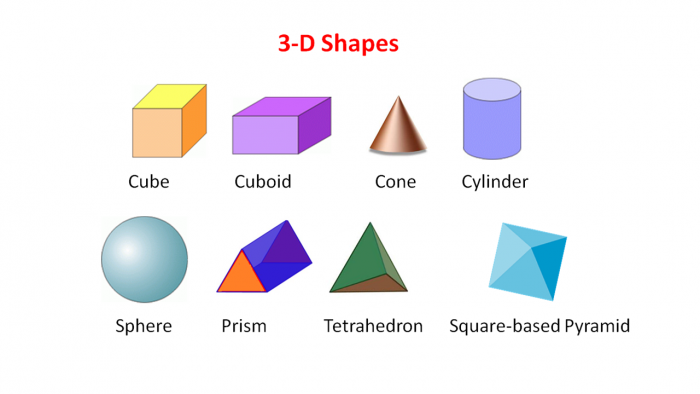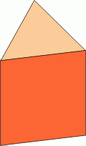# Investigate 3D Shapes

In this worksheet, students will be asked to use their knowledge of 3D shapes to investigate and solve a variety of worded problems.Key stage:  KS 2

Curriculum topic:   Geometry: Properties of Shapes

Curriculum subtopic:   Know 3D Shapes

Difficulty level:### QUESTION 1 of 10

In this activity, you will be asked to use your knowledge of 3D shapes to solve worded problems.

Let's recap the different shapes you may need to know about.Ready to get started?  Off we go!

Dylan thinks of a 3D shape.

He says... "It has six faces. Each face is a square."

Which shape is Dylan thinking of?

Cuboid

Cube

Triangular Prism

Sphere

Lucy thinks of a 3D shape.

She says, "The shape I'm thinking of has a total of five faces. One of the faces is a square, the other four faces are triangles.  Which shape am I thinking of?"

Hexagonal Prism

Triangular Prism

Cuboid

Square Based PyramidHow many vertices does the triangular prism have?

A different 3D shape (not a triangular prism) has eight vertices.

Which shape, out of the following, is it?

Square          Triangle           Cube          Cylinder

Can you match the description of the 3D shape to its correct name?

## Column B

Cube
six vertices, five faces
Square based pyramid
eight vertices, six faces
Triangular prism
four vertices, four faces
Triangular based pyramid
five vertices, five faces

Can you match each 3D shape to the correct number of faces?

## Column B

Cube
6
Cuboid
5
Hexagonal Prism
6
Triangular Prism
8

Can you choose the correct number of edges for each 3D shape?

 6 18 9 12 Cuboid Triangular Based Pyramid Triangular Prism Hexagonal Prism

Can you complete the blank spaces to describe and name the 3D shape?

 6 18 9 12 Cuboid Triangular Based Pyramid Triangular Prism Hexagonal Prism

Can you complete the blank spaces to describe and name the 3D shape?

 6 18 9 12 Cuboid Triangular Based Pyramid Triangular Prism Hexagonal Prism

When Max is drawing the faces of a cone, he draws one face.

Is he correct or incorrect to draw one face?

Correct

Incorrect

• Question 1

Dylan thinks of a 3D shape.

He says... "It has six faces. Each face is a square."

Which shape is Dylan thinking of?

Cube
EDDIE SAYS
The 3-D shape Dylan is thinking of is a cube, every side is a square and it has six faces.
• Question 2

Lucy thinks of a 3D shape.

She says, "The shape I'm thinking of has a total of five faces. One of the faces is a square, the other four faces are triangles.  Which shape am I thinking of?"

Square Based Pyramid
EDDIE SAYS
Did you work out which shape Lucy was talking about? Lucy's shape was a square based pyramid, it has five faces, a square base and then four triangular faces that meet to form a pyramid.
• Question 3How many vertices does the triangular prism have?

6
six
EDDIE SAYS
The triangular prism has six vertices. Remember, vertices are the points where two or more edges meet. This should all be pretty simple! Let's continue.
• Question 4

A different 3D shape (not a triangular prism) has eight vertices.

Which shape, out of the following, is it?

Square          Triangle           Cube          Cylinder

EDDIE SAYS
A cube has got six identical faces, each is a square. Therefore, it has eight vertices.
• Question 5

Can you match the description of the 3D shape to its correct name?

## Column B

Cube
eight vertices, six faces
Square based pyramid
five vertices, five faces
Triangular prism
six vertices, five faces
Triangular based pyramid
four vertices, four faces
EDDIE SAYS
How did you find matching each description with the correct label? This is all great practise. Cube = eight vertices and six faces. Square based pyramid = five vertices and five faces. Triangular prism = six vertices and five faces. Triangular based pyramid = four vertices and four faces.
• Question 6

Can you match each 3D shape to the correct number of faces?

## Column B

Cube
6
Cuboid
6
Hexagonal Prism
8
Triangular Prism
5
EDDIE SAYS
This question requires you to mentally picture each 3-D shape carefully, to accurately count the number of faces. Cube = six faces. Cuboid = six faces. Hexagonal prism = eight faces. Triangular prism = five faces.
• Question 7

Can you choose the correct number of edges for each 3D shape?

 6 18 9 12 Cuboid Triangular Based Pyramid Triangular Prism Hexagonal Prism
EDDIE SAYS
That was a tricky question. The total amount of edges for each 3D shape are: Cuboid = 12 Triangular based pyramid = 6 Triangular prism = 9 Hexagonal prism = 18
• Question 8

Can you complete the blank spaces to describe and name the 3D shape?

EDDIE SAYS
Hopefully, the fact this shape has a curved face and two flat additional faces gave enough clues! It's a cylinder! Three faces in total, one curved and two flat circles.
• Question 9

Can you complete the blank spaces to describe and name the 3D shape?

EDDIE SAYS
This time the 3-D shape was a cuboid as it has six faces, two square and four rectangular. It also has twelve edges and eight vertices. Getting easier?
• Question 10

When Max is drawing the faces of a cone, he draws one face.

Is he correct or incorrect to draw one face?

Correct
EDDIE SAYS
Max is correct. A cone only has one face! It has a curved face and a circle as its base. That's another activity completed! Why not take a short break then attempt another one?
---- OR ----